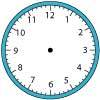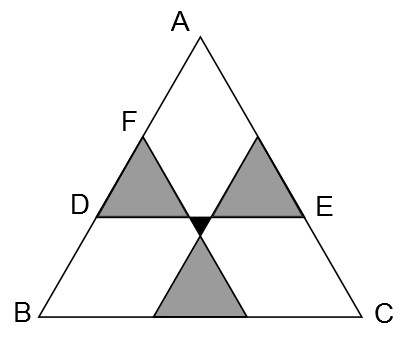#### You may also like### Right Time

At the time of writing the hour and minute hands of my clock are at right angles. How long will it be before they are at right angles again?### Isosceles

Prove that a triangle with sides of length 5, 5 and 6 has the same area as a triangle with sides of length 5, 5 and 8. Find other pairs of non-congruent isosceles triangles which have equal areas.Four rods, two of length a and two of length b, are linked to form a kite. The linkage is moveable so that the angles change. What is the maximum area of the kite?As triangle ABC is equilateral, ∠BAC = $60$°. Since the grey triangles are equilateral, ∠ADE = $60$°, so the triangle ADE is equilateral.
The length of the side of this triangle is equal to the length of $DE = (5 + 2 + 5)\;\mathrm{cm} = 12\;\mathrm{cm}$. So $AF = AD - FD = (12 - 5)\;\mathrm{cm} = 7\;\mathrm{cm}$.
By a similar argument, we deduce that $BD = 7\;\mathrm{cm}$, so the length of the side of the triangle $ABC = (7 + 5 + 7)\;\mathrm{cm} = 19\;\mathrm{cm}$.